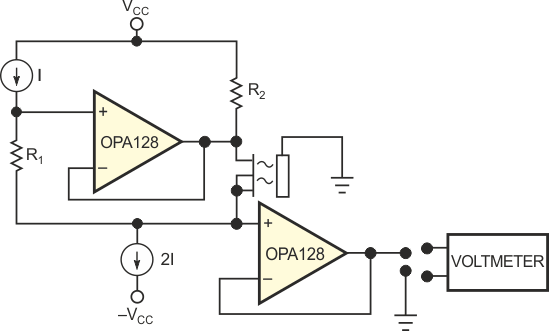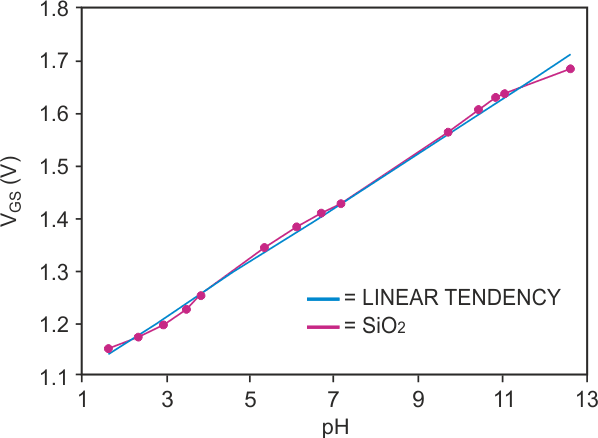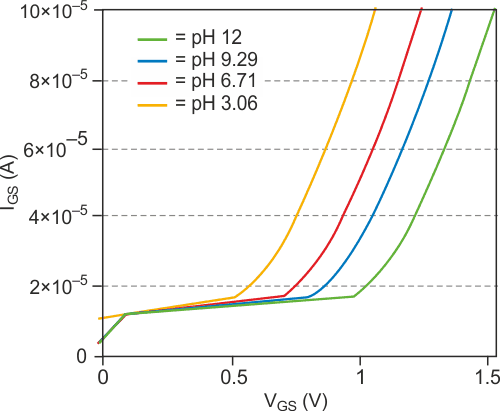# Circuit provides constant current for ISFETs/MEMFETs

## Texas Instruments OPA128 REF200

ISFETs and MEMFETs (ion-sensitive and membrane FETs) are solid-state chemical sensors that provide an electrical signal associated with the change of a chemical concentration in a solution. These types of sensors need a stable operating point. A common operating point is a drain-source current of 100 µA and a drain-source voltage of 500 mV. Under these conditions, the sensed chemical information is contained in the gate-source voltage, VGS. Figure 1 shows a constant-current driver for ISFETs/MEMFETs. The current source, I, produces a voltage drop in resistor R1; the voltage follower reflects this voltage to the drain-source terminals of the chemical sensor. In this example, VDS is equal to IR. The entire current, I, traverses resistor R1, because the op amp is an electrometer type with femtoamp-range input-bias current. The sensor's drain-source current, IGS, is the difference between the constant currents, 2I and I – in this case, I. A REF200 provides the constant currents in the circuit in Figure 1. The REF200 has two internal 100-µA current sources and a current mirror.Figure 1. A constant-current driver provides the basis for a chemical-concentration test system.Figure 2. The gate-source voltage shows good correlation with the concentration of [H1] ions.

One of the 100-µA current sources provides I in Figure 1. The lower 2I current source uses the REF200's second 100-µA current source and the internal current mirror. The second voltage follower measures the gate-source voltage of the sensor – hence, the chemical concentration of interest. Figure 2 shows experimental results. You can see that the setup provides a linear relationship between gate-source voltage and the concentration of [H+] ions. The experiment used SiO2 – and Si3N4 -based ISFET sensors. Figure 3 shows the variation of the threshold voltage as a function if pH.Figure 3. The gate-source voltage of an ISFET shifts with the degree of acidity.

## Materials on the topic

EDN